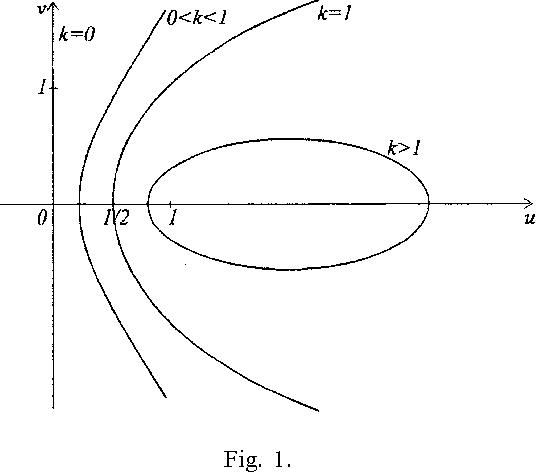You are currently offline. Some features of the site may not work correctly.

# Uniformly convex space

Known as: Uniform convexity, Uniformly convex Banach space
In mathematics, uniformly convex spaces (or uniformly rotund spaces) are common examples of reflexive Banach spaces. The concept of uniform convexity… Expand
Wikipedia

## Papers overview

Semantic Scholar uses AI to extract papers important to this topic.
Highly Cited
2008
Highly Cited
2008
We propose the class of uniformly convex \$W\$-hyperbolic spaces with monotone modulus of uniform convexity (\$UCW\$-hyperbolic… Expand
Highly Cited
1999
Highly Cited
1999
• 1999
• Corpus ID: 28830020
Abstract Let Ω k ⊂ C denote a domain, such that 1∈Ω k and ∂Ω k is a conic section, with eccentricity equal to 1/k. In this paper… Expand
•Highly Cited
1993
Highly Cited
1993
We investigate starlike functions f(z) = z + Yfk=2akzk wim the property that zf'(z)/'f(z) lies inside a certain parabola. These… Expand
Highly Cited
1991
Highly Cited
1991
• 1991
• Corpus ID: 122171983
Abstract Let X be a real Banach space with dual X ∗ and moduli of convexity and smoothness δ X ( e ) and ϱ X ( τ ), respectively… Expand
Highly Cited
1991
Highly Cited
1991
We introduce a new class of normalized functions regular and univalent in the unit disk. These functions, called uniformly convex… Expand
Highly Cited
1984
Highly Cited
1974
Highly Cited
1974
• 1974
• Corpus ID: 59414843
There is a uniformly convex Banach space with unconditional basis which contains no subspace isomorphic to any lp (1 p ~). The… Expand
Highly Cited
1955
Highly Cited
1955
which we shall call local uniform convexity. Geometrically this differs from uniform convexity in that it is required that one… Expand
Highly Cited
1953
Highly Cited
1953
(b) Show that if X is uniformly convex and (xn) ⊂ X such that lim n→∞ ‖xn‖ = 1 and lim n,m→∞ ‖xn + xm‖ = 2, then (xn) is… Expand
Highly Cited
1941
Highly Cited
1941
Let {Bi,i = l, 2, • . . } be a sequence of Banach spaces, and define B = P\Bi} to be the space of sequences b={bi} with biÇ^Bi… Expand